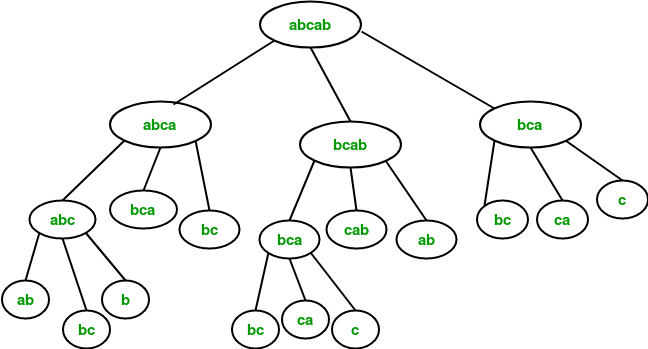# Recursive solution to count substrings with same first and last characters

• Difficulty Level : Easy
• Last Updated : 18 Jul, 2022

We are given a string S, we need to find count of all contiguous substrings starting and ending with same character.

Examples :

```Input  : S = "abcab"
Output : 7
There are 15 substrings of "abcab"
a, ab, abc, abca, abcab, b, bc, bca
bcab, c, ca, cab, a, ab, b
Out of the above substrings, there
are 7 substrings : a, abca, b, bcab,
c, a and b.

Input  : S = "aba"
Output : 4
The substrings are a, b, a and aba```

We have discussed different solutions in below post.
Count substrings with same first and last characters

In this article, a simple recursive solution is discussed.

Implementation:

## C++

 `// c++ program to count substrings with same``// first and last characters``#include ``#include ``using` `namespace` `std;` `/* Function to count substrings with same first and``  ``last characters*/``int` `countSubstrs(string str, ``int` `i, ``int` `j, ``int` `n)``{``    ``// base cases``    ``if` `(n == 1)``        ``return` `1;``    ``if` `(n <= 0)``        ``return` `0;` `    ``int` `res =  countSubstrs(str, i + 1, j, n - 1) + ``               ``countSubstrs(str, i, j - 1, n - 1) -``               ``countSubstrs(str, i + 1, j - 1, n - 2);           ` `    ``if` `(str[i] == str[j])``        ``res++;` `    ``return` `res;``}` `// driver code``int` `main()``{``    ``string str = ``"abcab"``;``    ``int` `n = str.length();``    ``cout << countSubstrs(str, 0, n - 1, n);``}`

## Java

 `// Java program to count substrings``// with same first and last characters` `class` `GFG``{``    ``// Function to count substrings``    ``// with same first and``    ``// last characters``    ``static` `int` `countSubstrs(String str, ``int` `i,``                                         ``int` `j, ``int` `n)``    ``{``        ``// base cases``        ``if` `(n == ``1``)``            ``return` `1``;``        ``if` `(n <= ``0``)``            ``return` `0``;``    ` `        ``int` `res = countSubstrs(str, i + ``1``, j, n - ``1``) +``                ``countSubstrs(str, i, j - ``1``, n - ``1``) -``                ``countSubstrs(str, i + ``1``, j - ``1``, n - ``2``);        ``    ` `        ``if` `(str.charAt(i) == str.charAt(j))``            ``res++;``    ` `        ``return` `res;``    ``}``    ` `    ``// Driver code``    ``public` `static` `void` `main (String[] args)``    ``{``        ``String str = ``"abcab"``;``        ``int` `n = str.length();``        ``System.out.print(countSubstrs(str, ``0``, n - ``1``, n));``    ``}``}` `// This code is contributed by Anant Agarwal.`

## Python3

 `# Python 3 program to count substrings with same``# first and last characters` `# Function to count substrings with same first and``# last characters``def` `countSubstrs(``str``, i, j, n):` `    ``# base cases``    ``if` `(n ``=``=` `1``):``        ``return` `1``    ``if` `(n <``=` `0``):``        ``return` `0` `    ``res ``=` `(countSubstrs(``str``, i ``+` `1``, j, n ``-` `1``)``        ``+` `countSubstrs(``str``, i, j ``-` `1``, n ``-` `1``)``        ``-` `countSubstrs(``str``, i ``+` `1``, j ``-` `1``, n ``-` `2``))    ` `    ``if` `(``str``[i] ``=``=` `str``[j]):``        ``res ``+``=` `1` `    ``return` `res` `# driver code``str` `=` `"abcab"``n ``=` `len``(``str``)``print``(countSubstrs(``str``, ``0``, n ``-` `1``, n))` `# This code is contributed by Smitha`

## C#

 `// C# program to count substrings``// with same first and last characters``using` `System;` `class` `GFG {``    ` `    ``// Function to count substrings``    ``// with same first and``    ``// last characters``    ``static` `int` `countSubstrs(``string` `str, ``int` `i,``                                 ``int` `j, ``int` `n)``    ``{``        ` `        ``// base cases``        ``if` `(n == 1)``            ``return` `1;``        ``if` `(n <= 0)``            ``return` `0;``    ` `        ``int` `res = countSubstrs(str, i + 1, j, n - 1)``                ``+ countSubstrs(str, i, j - 1, n - 1)``            ``- countSubstrs(str, i + 1, j - 1, n - 2);    ``    ` `        ``if` `(str[i] == str[j])``            ``res++;``    ` `        ``return` `res;``    ``}``    ` `    ``// Driver code``    ``public` `static` `void` `Main ()``    ``{``        ``string` `str = ``"abcab"``;``        ``int` `n = str.Length;``        ` `        ``Console.WriteLine(``                ``countSubstrs(str, 0, n - 1, n));``    ``}``}` `// This code is contributed by vt_m.`

## PHP

 ``

## Javascript

 ``

Output

`7`

The time complexity of above solution is exponential. In Worst case, we may end up doing O(3n) operations.

Auxiliary Space:  O(n), where n is the length of string.

This is because when string is passed in the function it creates a copy of itself in stack.There is also a divide and conquer recursive approach

The idea is to split the string in half until we get one element and have our base case return 2 things

1. a map containing the character to the number of occurrences (i.e a:1 since its the base case)
2. (This is the total number of substring with start and end with the same char. Since the base case is a string of size one, it starts and ends with the same character)

Then combine the solutions of the left and right division of the string, then return a new solution based on left and right. This new solution can be constructed by multiplying the common characters between the left and right set and adding to the solution count from left and right, and returning a map containing element occurrence count and solution count

Implementation:

## Python3

 `# code``def` `countSubstr(s):``    ``if` `len``(s) ``=``=` `0``:``        ``return` `0``    ``charMap, numSubstr ``=` `countSubstrHelper(s, ``0``, ``len``(s)``-``1``)``    ``return` `numSubstr`  `def` `countSubstrHelper(string, start, end):``    ``if` `start >``=` `end:  ``# our base case for the recursion. When we have one character``        ``return` `{string[start]: ``1``}, ``1``    ``mid ``=` `(start ``+` `end)``/``/``2``    ``mapLeft, numSubstrLeft ``=` `countSubstrHelper(``        ``string, start, mid)  ``# solve the left half``    ``mapRight, numSubstrRight ``=` `countSubstrHelper(``        ``string, mid``+``1``, end)  ``# solve the right half``    ``# add number of substrings from left and right``    ``numSubstrSelf ``=` `numSubstrLeft ``+` `numSubstrRight` `    ``# multiply the characters from left set with matching characters from right set``    ``# then add to total number of substrings``    ``for` `char ``in` `mapLeft:``        ``if` `char ``in` `mapRight:``            ``numSubstrSelf ``+``=` `mapLeft[char] ``*` `mapRight[char]` `    ``# Add all the key,value pairs from right map to left map``    ``for` `char ``in` `mapRight:``        ``if` `char ``in` `mapLeft:``            ``mapLeft[char] ``+``=` `mapRight[char]``        ``else``:``            ``mapLeft[char] ``=` `mapRight[char]``    ``# Return the map of character and the sum of substring from left, right and self``    ``return` `mapLeft, numSubstrSelf`  `print``(countSubstr(``"abcab"``))` `# Contributed by Xavier Jean Baptiste`

Output

`7`

The time complexity of the above solution is O(nlogn) with space complexity O(n) which occurs if all elements are distinct

This article is contributed by Yash Singla. If you like GeeksforGeeks and would like to contribute, you can also write an article using write.geeksforgeeks.org or mail your article to review-team@geeksforgeeks.org. See your article appearing on the GeeksforGeeks main page and help other Geeks.

My Personal Notes arrow_drop_up